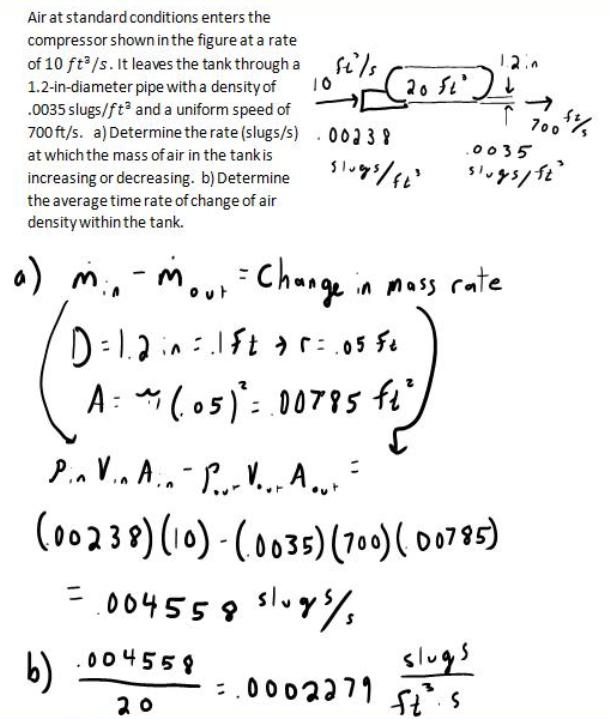fluid mechanics math problems engineering equations fluid mechanics basic mechanics school homework engineering math fluids formulas fluid mechanics problems fluid mechanics problem solutions to fluids problems full solution fluid mechanics engineering fluids problem solution fluid mechanics math problems engineering equations fluid mechanics basic mechanics school homework engineering math fluid mechanics formulas fluid problems fluid mechanics problem solutions to fluid mechanics problems full solution fluid mechanics engineering fluid mechanics problem solution
fluid mechanics math problems engineering equations fluid mechanics basic mechanics school homework engineering math fluids formulas fluid mechanics problems fluid mechanics problem solutions to fluids problems full solution fluid mechanics engineering fluids problem solution fluid mechanics math problems engineering equations fluid mechanics basic mechanics school homework engineering math fluid mechanics formulas fluid problems fluid mechanics problem solutions to fluid mechanics problems full solution fluid mechanics engineering fluid mechanics problem solution
Highalphabet Home Page fluid mechanics solutions fluids math solved Fluid Mechanics Page 1
Air at standard conditions enters the compressor shown in the figure at a rate of 10 ft^3/s. It leaves the tank through a 1.2 in diameter pipe with a density of .0035 slugs/ft^3 and a uniform speed of 700 ft/s. a) Determine the rate (slugs/s) at which the mass of the air in the tank is increasing or decreasing. b) Determine the average time rate of change of air densityh within the tank.Air at standard conditions enters the compressor shown in the figure at a rate of 10 ft^3/s. It leaves the tank through a 1.2 in diameter pipe with a density of .0035 slugs/ft^3 and a uniform speed of 700 ft/s. a) Determine the rate (slugs/s) at which the mass of the air in the tank is increasing or decreasing. b) Determine the average time rate of change of air densityh within the tank.